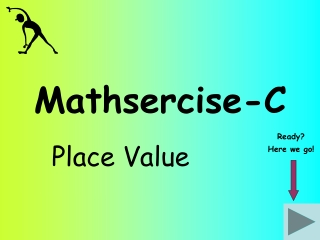DownloadDownload PresentationMathsercise-C

# Mathsercise-C

Télécharger la présentation## Mathsercise-C

- - - - - - - - - - - - - - - - - - - - - - - - - - - E N D - - - - - - - - - - - - - - - - - - - - - - - - - - -
##### Presentation Transcript

1. Mathsercise-C Place Value Ready? Here we go!

2. Using the information that 14 x 23 = 322 Write down the value of.. Question 2 (i) 1.4 x 2.3 1.4 x 23 = 32.2 This question has both values with one decimal point movement Notice that the decimal point has moved the other way when you divide! Re-arrange the original calculation first! 1.4 x 2.3 = 3.22 (ii) 322 ÷ 2.3 Answer 322 ÷ 23 = 14 1 322 ÷ 2.3 = 140 Place Value

3. Using the information that 58 x 117 = 6786 Write down the value of.. (i) 0.58 x 117,000 Question 3 5.8 x 117 = 678.6 0.58 x 117 = 67.86 0.58 x 117,000 = 67,860 Notice which way the decimal point moves! Answer (ii) 67.86 ÷ 5.8 6786 ÷ 58 = 117 67.86 ÷ 58 = 1.17 2 Place Value 67.86 ÷ 5.8 = 11.7

4. Using the information that 11 x 19 = 209 To find the Lowest Common Multiple (LCM) of 33 and 19 Question 4 33 is 3 x 11 Answer So…the LCM of 33 and 19 is 3 x 11 x 19… or…3 x 209 = 627 3 Place Value

5. Using the information that 39 x 17 = 663 To find the Lowest Common Multiple (LCM) of 13 and 17 Start As 39 ÷ 3 is 13 then LCM of 13 and 17 is 663 ÷ 3 = 221 Answer 4 Place Value